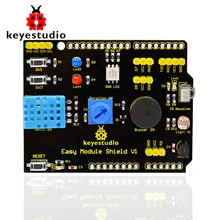Home Chipkit Chipkit and keyestudio Easy module Shield V1

# Chipkit and keyestudio Easy module Shield V1

On to another Arduino shield and this is one of my favourites, its a low cost shield which contains a variety of resources on it making it ideal for beginners. We will again connect this to a Chipkit Max32 and the examples will be written in the arduino IDE

here is a picture of the shield### Features

You can see most of the features but here is a quick summary of what is fitted to the shield

two LEDs
two switches
reset button
Measuring temperature and humidity with DHT11 temperature and humidity sensor
passive buzzer module
Full-color RGB LED
Utilizing photovaristor to detect the brightness of light
LM35D temperature sensor
2-channel digital port(D7.D8)
1-channel analog port(A3)

### Code Examples

None of these examples need any external libraries,

Button example 1

 Source code```const int buttonPin = 3;     // the number of the pushbutton pin
const int ledPin =  12;      // the number of the LED pin

int buttonState = 0;         // variable for reading the pushbutton status

void setup()
{
// initialize the LED pin as an output:
pinMode(ledPin, OUTPUT);
pinMode(buttonPin, INPUT);
}

void loop()
{
// read the state of the pushbutton value:
// check if the pushbutton is pressed.
// if it is, the buttonState is LOW:
if (buttonState == LOW)
{
// turn LED on:
digitalWrite(ledPin, HIGH);
}
else
{
// turn LED off:
digitalWrite(ledPin, LOW);
}
}```

Button example 2

 Source code```const int button1Pin = 2;     // the number of the pushbutton pin
const int button2Pin = 3;
const int led1Pin =  12;      // the number of the LED pin
const int led2Pin =  13;

int button1State = 0;         // variable for reading the pushbutton status
int button2State = 0;

void setup()
{
// initialize the LED pin as an output:
pinMode(led1Pin, OUTPUT);
pinMode(led2Pin, OUTPUT);
pinMode(button1Pin, INPUT);
pinMode(button2Pin, INPUT);
}

void loop()
{
// read the state of the pushbutton value:

// check if the pushbutton is pressed.
// if it is, the buttonState is LOW:
if (button1State == LOW)
{
digitalWrite(led1Pin, HIGH);
}
else
{
digitalWrite(led1Pin, LOW);
}

// read the state of the pushbutton value:

// check if the pushbutton is pressed.
// if it is, the buttonState is LOW:
if (button2State == LOW)
{
digitalWrite(led2Pin, HIGH);
}
else
{
digitalWrite(led2Pin, LOW);
}
}```

LED example

 Source code```#define redLed 12
#define blueLed 13

void setup()
{
pinMode(redLed, OUTPUT);
pinMode(blueLed, OUTPUT);
}

void loop()
{
digitalWrite(redLed, HIGH);   // turn the LED on (HIGH is the voltage level)
digitalWrite(blueLed, LOW);    // turn the LED off by making the voltage LOW
delay(1000);
digitalWrite(redLed, LOW);    // turn the LED off by making the voltage LOW
digitalWrite(blueLed, HIGH);   // turn the LED on (HIGH is the voltage level)
delay(1000);
}```

POT example

 Source code```#define vrPin A0

void setup()
{
// initialize serial communication at 9600 bits per second:
Serial.begin(9600);
}

void loop()
{
// read the variable resistor on analog pin 0:
// Convert the analog reading to a voltage
float vrVoltage = vrValue * (5.0 / 1023.0);
// print out the Variable resistor value you read:
Serial.print("Variable resistor : ");
Serial.println(vrVoltage);
delay(1000);
}```

RGB led example

 Source code```#define redLed 9
#define greenLed 10
#define blueLed 11

void setup()
{

pinMode(redLed, OUTPUT);
pinMode(greenLed, OUTPUT);
pinMode(blueLed, OUTPUT);
}

void loop()
{
for (int i=0; i <= 255; i++)
{
setColor(i, 0, 0);  // red
delay(10);
}
}

void setColor(int red, int green, int blue)
{
analogWrite(redLed, red);
analogWrite(greenLed, green);
analogWrite(blueLed, blue);
}```